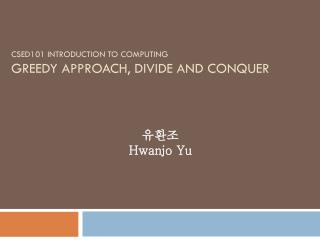Download PresentationCSED101 Introduction to Computing greedy approach, divide and conquer

# CSED101 Introduction to Computing greedy approach, divide and conquer - PowerPoint PPT PresentationDownload Presentation## CSED101 Introduction to Computing greedy approach, divide and conquer

- - - - - - - - - - - - - - - - - - - - - - - - - - - E N D - - - - - - - - - - - - - - - - - - - - - - - - - - -
##### Presentation Transcript

1. 유환조 Hwanjo Yu CSED101 Introduction to Computinggreedy approach, divide and conquer

2. Design of Algorithm • Computing problem • Given inputs, how to generate the desirable outputs? • Algorithm • Sequence of instructions or process to solve a computing problem • Design of algorithm • There could be many ways to solve a computing problem. • Each way may take a different amount of time and generate different results

3. Greedy approach • When designing an algorithm, greedy approach does NOT look at the problem in a holistic way. Instead, it just follows the LOCALLY optimal way at each step. Thus, it may NOT find GLOBALLY OPTIMAL solution. • E.g.,finding the shortest path from Pohang to Seoul • To find the optimal (or the shortest) path, one needs to compute the distance of every possible path from Pohang to Seoul. • Greedy approach selects the shortest way to Seoul at each step.

4. Shortest path from Pohang to Seoul Pohang Seoul Found path = optimal path

5. Shortest path from Pohang to Seoul Optimal path Pohang Seoul better path Found path

6. Coin problem • We want to minimize the number of coins to make a certain amount of money • We have three types of coins - 100, 50, and 10 won. • We want to make 160 wons • Greedy approach: Use the largest coin first that does not exceed the target money • Select one 100 • Select one 50 • Select one 10 • Total three coins => Optimal

7. Coin problem • What if the three types of coins we have are 120, 50, and 10 wons? • Greedy approach to make 160 wons • Select one 120 • Select four 10 • Total five coins we consumed. • The optimal solution in this case is three 50 and one 10 wons => total four coins consumed

8. Conclusion: Greedy approach • Greedy approach • Normally find a good solution which is sometimes optimal and sometimes not

9. Divide and conquer • Divide a big problem into several small problems • Induce the solution of the big problem from solutions of small problems

10. Tower of Hanoi • The objective of the puzzle is to move the entire stack to another rod, obeying the following rules: • Only one disk may be moved at a time. • Each move consists of taking the upper disk from one of the pegs and sliding it onto another rod, on top of the other disks that may already be present on that rod. • No disk may be placed on top of a smaller disk. a b c

11. Tower of Hanoi • Problem: Move n disks • Move n-1 disks from a to b • Move the biggest disk from a to c • Move n-1 disks from b to c • Problem: Move n-1 disks • Move n-2 disks … a b c

12. Tower of Hanoi • Divide and conquer • Moving n disks is divided into moving n-1 disks twice. • Moving n-1 disks is divided into moving n-2 disks twice. • … • Moving 1 disk is simple a b c

13. Tower of Hanoi • Simple solution • Alternate moves between the smallest piece and a non- smallest piece. • When moving the smallest piece, always move it in the same direction (either to the left or to the right, but be consistent). If there is no tower in the chosen direction, move it to the opposite end. When the turn is to move the non-smallest piece, there is only one legal move. a b c

14. Divide and conquer • Divide-and-conquer is a widely used principle in algorithm design • “Dynamic programming” is an implementation method using recursive function to implement the divide-and-conquer. • Recursive function is based on the same principle of the divide-and-conquer. • Tail-recursive function is an efficient way to implement the divide-and-conquer.

15. Next week • Sorting • Greedy approach • Selection sort • Insertion sort • Divide-and-conquer • Merge sort • Quick sort